# Depth-first search traversal in Javascript

DFS visits the child vertices before visiting the sibling vertices; that is, it traverses the depth of any particular path before exploring its breadth. A stack (often the program's call stack via recursion) is generally used when implementing the algorithm.

Following is how a DFS works −

• Visit the adjacent unvisited vertex. Mark it as visited. Display it. Push it in a stack.
• If no adjacent vertex is found, pop up a vertex from the stack. (It will pop up all the vertices from the stack, which do not have adjacent vertices.)
• Repeat Rule 1 and Rule 2 until the stack is empty.

Let us look at an illustration of how DFS traversal works.

StepTraversalDescription
1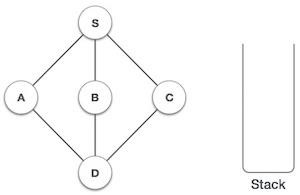Initialize the stack.
2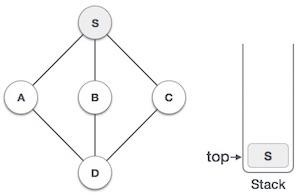Mark S as visited and put it onto the stack. Explore any unvisited adjacent node from S. We have three nodes and we can pick any of them. For this example, we shall take the node in an alphabetical order.
3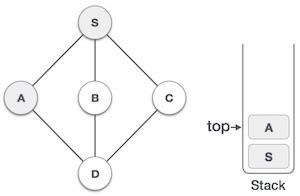Mark A as visited and put it onto the stack. Explore any unvisited adjacent node from A. Both S and D are adjacent to A but we are concerned for unvisited nodes only.
4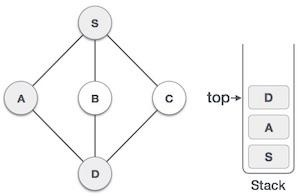Visit D and mark it as visited and put onto the stack. Here, we have B and Cnodes, which are adjacent to Dand both are unvisited. However, we shall again choose in an alphabetical order.
5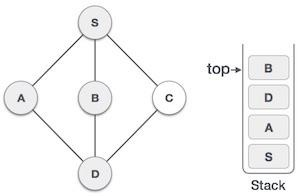We choose B, mark it as visited and put onto the stack. Here B does not have any unvisited adjacent node. So, we pop B from the stack.
6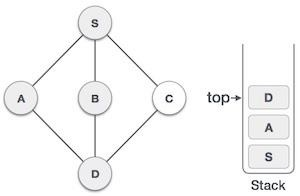We check the stack top for a return to the previous node and check if it has any unvisited nodes. Here, we find D to be on the top of the stack.
7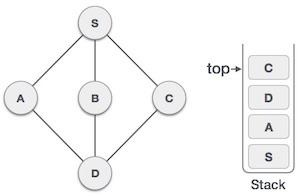Only unvisited adjacent node is from D is Cnow. So we visit C, mark it as visited and put it onto the stack.

As C does not have any unvisited adjacent node so we keep popping the stack until we find a node that has an unvisited adjacent node. In this case, there's none and we keep popping until the stack is empty. Let us look at how we can implement this in JavaScript.

## Example

DFS(node) {
// Create a Stack and add our initial node in it
let s = new Stack(this.nodes.length);
let explored = new Set();
s.push(node);

// Mark the first node as explored

// We'll continue till our Stack gets empty
while (!s.isEmpty()) {
let t = s.pop();

// Log every element that comes out of the Stack
console.log(t);

// 1. In the edges object, we search for nodes this node is directly connected to.
// 2. We filter out the nodes that have already been explored.
// 3. Then we mark each unexplored node as explored and push it to the Stack.
this.edges[t]
.filter(n => !explored.has(n))
.forEach(n => {
s.push(n);
});
}
}

Well, that was easy. We really just swapped the queue out for the stack and voila, we have DFS. That is really the only difference between the 2. DFS can also be implemented using recursion. But that is best avoided in this case as a bigger graph means we need extra memory just to keep track of the call stack.

You can test this using −

let g = new Graph();

g.DFS("A");

## Output

This will give the output.

A
D
E
F
B
G
C